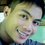# Euler's Phi Function of a Palindromic Prime

The Euler's Totient Function (phi function) φ of a palindromic prime p is easy to compute.

Definition of Terms:

Euler's Phi Function- a number of positive integers less than or equal to n that are relatively prime to n.

Palindrome- a word, a phrase, a sequence, or a number that reads the same backward as forward.

Prime- a natural number greater than 1 that has no positive divisors other than 1 and itself.

Palindromic prime- a number that is simultaneously palindromic and prime.

Conjecture:

The phi function φ of every palindromic prime p is equal to a given palindromic prime p minus 1.

In this conjecture, let n equals p.

Note: Palindromic prime, prime palindrome and palprime are synonymous. 🙋

In symbols:

φ(p)= p-1 (FORMULA) 👈👦

Example 1:

p= 929 (a palindromic prime)

Solution:

Use the formula.

φ(929) = 929-1

= 928 ✔👍


Hence, φ(929) = 928.

Example 2:

p= 13,331 (a palindromic prime)

Solution:

Use the formula.

 φ(13331) = 13331-1

= 13,330 ✔👍


Hence, φ(13331) = 13,330.

Now, you try! 😊

Exercises 📚

Compute the following:

1. φ(10301)

2. φ(1411141)

3. φ(7619167)

4. φ(7630367)

5. φ(9989899)


Author: John Paul L. Hablado, LPT

     (c) April 11, 2017


References:

Kindly click each example link for the URL (Uniform Resource Locator), and some related theorems on Euler's Phi Function of a Palindromic Prime. ❤4 years, 3 months ago

This discussion board is a place to discuss our Daily Challenges and the math and science related to those challenges. Explanations are more than just a solution — they should explain the steps and thinking strategies that you used to obtain the solution. Comments should further the discussion of math and science.

When posting on Brilliant:

• Use the emojis to react to an explanation, whether you're congratulating a job well done , or just really confused .
• Ask specific questions about the challenge or the steps in somebody's explanation. Well-posed questions can add a lot to the discussion, but posting "I don't understand!" doesn't help anyone.
• Try to contribute something new to the discussion, whether it is an extension, generalization or other idea related to the challenge.

MarkdownAppears as
*italics* or _italics_ italics
**bold** or __bold__ bold
- bulleted- list
• bulleted
• list
1. numbered2. list
1. numbered
2. list
Note: you must add a full line of space before and after lists for them to show up correctly
paragraph 1paragraph 2

paragraph 1

paragraph 2

[example link](https://brilliant.org)example link
> This is a quote
This is a quote
    # I indented these lines
# 4 spaces, and now they show
# up as a code block.

print "hello world"
# I indented these lines
# 4 spaces, and now they show
# up as a code block.

print "hello world"
MathAppears as
Remember to wrap math in $$ ... $$ or $ ... $ to ensure proper formatting.
2 \times 3 $2 \times 3$
2^{34} $2^{34}$
a_{i-1} $a_{i-1}$
\frac{2}{3} $\frac{2}{3}$
\sqrt{2} $\sqrt{2}$
\sum_{i=1}^3 $\sum_{i=1}^3$
\sin \theta $\sin \theta$
\boxed{123} $\boxed{123}$